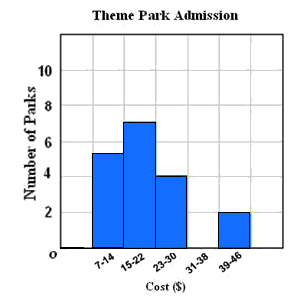# Histograms

A histogram is a type of bar graph used to display numerical data that has been organized into equal intervals. The bars should touch the adjacent bars but not overlaps. These intervals allow you to see the frequency distribution of the data, or how many pieces of data are in each interval.

Example:

The frequency table shows the admission costs of various theme parks. Display the data in a histogram.

 Costs(\$) Tally Frequency $7-14$ ///// $5$ $15-22$ ///// // $7$ $23-30$ //// $4$ $31-38$ $0$ $39-46$ // $2$

Step $1$ : Draw and label a horizontal and vertical axis as shown. Include the title.

Step $2$ : Show the intervals from the frequency table on the horizontal axis and an interval of $2$ units on the vertical axis.

Step $3$ : For each cost interval, draw a bar whose height is given by the frequency.